The OpenFOAM Foundation
geometricOneField Class Reference

A class representing the concept of a GeometricField of 1 used to avoid unnecessary manipulations for objects which are known to be one at compile-time. More...

Inheritance diagram for geometricOneField: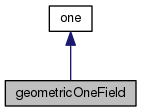[legend]
Collaboration diagram for geometricOneField:[legend]

## Public Types

typedef oneField Internal

typedef oneField Patch

typedef oneFieldField Boundary

typedef one cmptTypePublic Types inherited from one
typedef one value_type

## Public Member Functions

geometricOneField ()
Construct null. More...

const dimensionSetdimensions () const

one operator[] (const label) const

oneField field () const

oneField oldTime () const

Internal operator() () const

Internal v () const

Internal::FieldType primitiveField () const

Boundary boundaryField () constPublic Member Functions inherited from one
one ()
Construct null. More...

operator label () const
Return 1 for label. More...

operator float () const
Return 1 for float. More...

operator double () const
Return 1 for double. More...

## Detailed Description

A class representing the concept of a GeometricField of 1 used to avoid unnecessary manipulations for objects which are known to be one at compile-time.

Used for example as the density argument to a function written for compressible to be used for incompressible flow.

Definition at line 52 of file geometricOneField.H.

## Member Typedef Documentation

 typedef oneField Internal

Definition at line 61 of file geometricOneField.H.

 typedef oneField Patch

Definition at line 62 of file geometricOneField.H.

 typedef oneFieldField Boundary

Definition at line 63 of file geometricOneField.H.

 typedef one cmptType

Definition at line 64 of file geometricOneField.H.

## Constructor & Destructor Documentation

 geometricOneField ( )
inline

Construct null.

Definition at line 69 of file geometricOneField.H.

Here is the call graph for this function: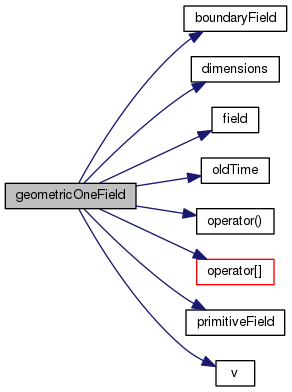## Member Function Documentation

 const Foam::dimensionSet & dimensions ( ) const
inline

Definition at line 30 of file geometricOneFieldI.H.

References Foam::dimless.

Referenced by geometricOneField::geometricOneField().

Here is the caller graph for this function: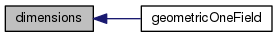Foam::one operator[] ( const label ) const
inline

Definition at line 36 of file geometricOneFieldI.H.

References one::one().

Referenced by geometricOneField::geometricOneField().

Here is the call graph for this function: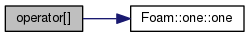Here is the caller graph for this function: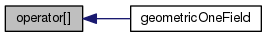Foam::oneField field ( ) const
inline

Definition at line 42 of file geometricOneFieldI.H.

Referenced by geometricOneField::geometricOneField().

Here is the caller graph for this function: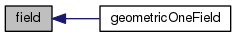Foam::oneField oldTime ( ) const
inline

Definition at line 48 of file geometricOneFieldI.H.

Referenced by geometricOneField::geometricOneField().

Here is the caller graph for this function: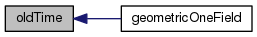Foam::geometricOneField::Internal operator() ( ) const
inline

Definition at line 55 of file geometricOneFieldI.H.

Referenced by geometricOneField::geometricOneField().

Here is the caller graph for this function: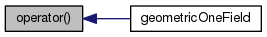Foam::geometricOneField::Internal v ( ) const
inline

Definition at line 62 of file geometricOneFieldI.H.

Referenced by geometricOneField::geometricOneField().

Here is the caller graph for this function: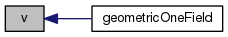Foam::geometricOneField::Internal::FieldType primitiveField ( ) const
inline

Definition at line 69 of file geometricOneFieldI.H.

Referenced by geometricOneField::geometricOneField().

Here is the caller graph for this function: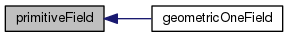Foam::geometricOneField::Boundary boundaryField ( ) const
inline

Definition at line 76 of file geometricOneFieldI.H.

Referenced by geometricOneField::geometricOneField().

Here is the caller graph for this function: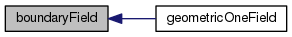The documentation for this class was generated from the following files: